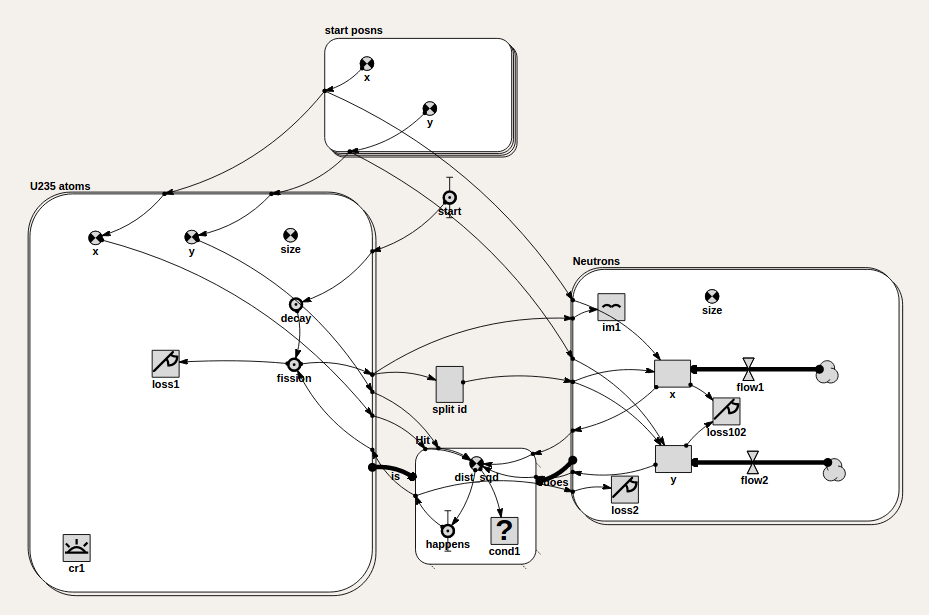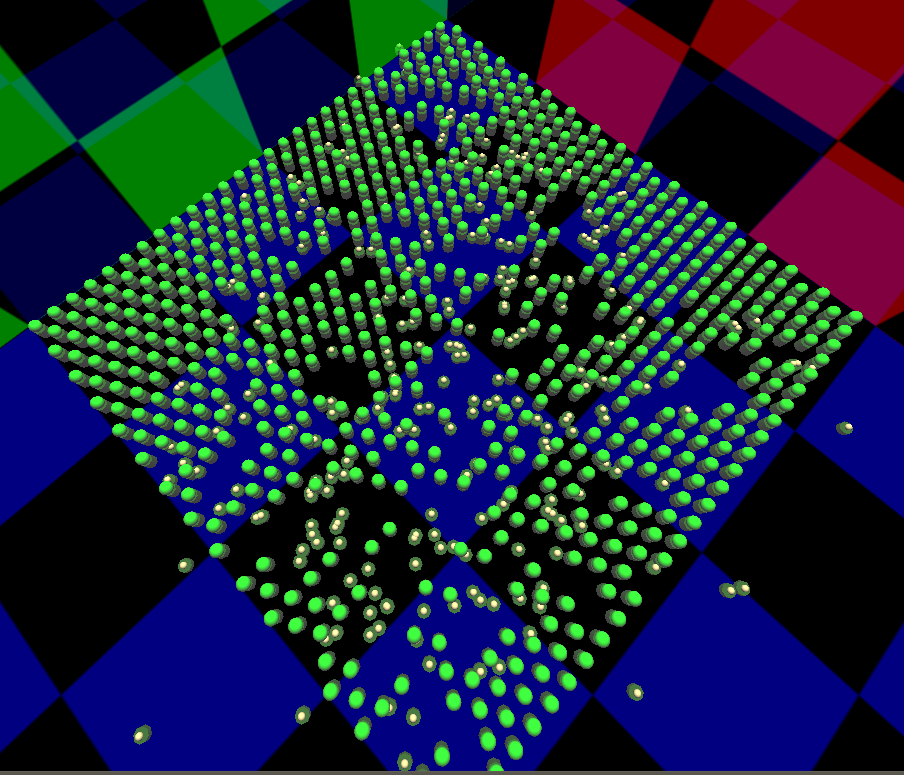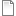# Nuclear fission - chain reaction

ModelId:
fission4.sml
SimileVersion:
6.6p2

This model simulates the process of nuclear fission by chain reaction, such as occurs in a fission reactor or weapon. It models a sample of a fissile material, such as plutonium, which has the property that when a nucleus is hit by a neutron it splits, releasing two more neutrons which are capable of splitting other nuclei. In a fission reactor this process is continuous, and regulated by the insertion of rods of a material that absorbs neutrons, in order to release a controllable amount of energy. In a nuclear weapon, two pieces of fissile material that separately have too large a surface area to volume ratio to sustain a chain reaction are brought together to create a single piece that exceeds the "critical mass". This then undergoes a runaway chain reaction releasing all the energy at once in an explosion.

The model uses a 2-D world rather than 3-D simply because it makes the process easier to visualize. The submodel on the left represents a hexagonal grid of atoms, but rather than using the hexagonal grid submodel it is a population where the individuals get their grid coordinates from another submodel. This is because we want to remove individual atoms as the simulation runs, and we want their indices to be numbers rather than vectors, so they can be made the values of discrete events. (Later versions of Simile allow vector-valued events).

The submodel on the right represents the neutrons. Initially there are none, but the fissile material also undergoes spontaneous radioactive decay, which produces neutrons. When an atom splits, the event is passed to the immigration channel for neutrons, causing two to be added. (Note that if population channels are driven from events, the exact number of individuals are added or removed immediately). Also a state variable outside the submodels is set to the index of the atom that split, so that when the new neutron-individuals are initialized they can get the position of the atom from the coordinate submodel to initialize their own positions. Their trajectory is set at random.

To handle splitting of atoms by neutrons, there is an association submodel between atoms and neutrons which has the existence condition that the distance between them is less than 1 unit. Inside this submodel is a limit event that triggers when the distance goes down to 0.5 unit. This event represents the actual splitting. It would be possible to have the existence condition be 0.5 unit and the event always true on initialization, but that would make the model behave differently. The existence of the association is only calculated on aregular time step boundary, so if the splitting condition was the same, all the splits would occur on time step boundaries, meaning several at once which would interfere with the mechanism for initializing neutron positions. Having the association come into existence before the actual split means that extrapolation is used to calculate the exact time of the split, so all splits occur separately. Unfortunately this makes the model run more slowly, since the whole model state is evaluated each time an event occurs.

The split event in the association is used to trigger the fission event in the corresponding atom, and to remove the neutron that does the splitting. The influences for these connections have been edited to disable the 'all values' interpretation, otherwise one split event would remove all neutrons and trigger fission in all atoms. Finally, neutrons are removed from the model if they move out of the lattice to speed up execution.

### Update -- base instance lookup

A new version of the model has been added, using base instance lookup to make it run faster. The problem with the original version is that, at the height of the chain reaction there will be many hundreds of neutrons and many hundreds of remaining atoms of fissile material. This means that the membership condition of the association submodel 'Hit' must be checked hundreds of thousands of times each time step, causing the model to slow down at this point. The problem is especially severe because each event counts as a time step boundary, and fission events are much more frequent than the periodic time step at this point.

The solution is base instance lookup, also known as one-sided relation enumeration. Instead of checking every neutron's position against that of every fissile atom, we make use of the fact that the atoms' positions are fixed and regular to tell which one a neutron is closest to, then we need only check for colission with that atom. To do this we add 'col' and 'row' variables to the neutron submodel, with influences from the X and Y compartments respectively. The equations are

col = round(X/3)

row = round(Y/3.464 - 0.25)

These reverse the calculations done in the 'start posns' submodel to derive the atom positions from the index number. Now we add influences from these variables to the condition in the 'Hit' submodel, and set the condition's equation to

index(1) is 32*(row-1)+col

The use of 'is' rather than '==' in the condition means that rather than comparing the index of each individual atom with that generated from the neutron position, we should simply pick the atom with that index. Note we no longer need an influence to the condition from 'dist sqd'.

Finally we need to check 'allow base instance lookup' in the properties of the role arrow 'is' connecting the atom model to the Hit model. This ensures that index(1) in the Hit model (which can be looked up) corresponds to the index of the atom individual. Because the atoms are a population, there is still an iteration involved in finding the one with the given index (if it still exists) but this is quicker than calculating the distance for each atom.Equations:

Model Desktop1 :

Event   start :
start =         1 (boolean)
Maximum = 0
Event that occurs once at start of simulation

State   split id :
split id =         [[U235 atoms/fission,trigger_magnitude()],[reset...,0]] (real)

Only one atom should split at a time, so this state is the index of the last atom to split

Submodel Hit :

Submodel "Hit" an association submodel between "U235 atoms" (in role "is") and "Neutrons" (in role "does").

Condition   cond1 :
cond1 =         dist<1 (cond_spec)
Where:
dist = Value(s) of dist_sqd

True if neutron and atom are less than 1 distance unit apart. This allows the time of the collision event to be set precisely since the association will already exist.

Event   happens :
happens =         dist_sqd (boolean)
Where:
dist_sqd = Value(s) of dist_sqd
Minimum = 0.25
Splitting happens if neutron gets within 0.5 distance units of atom.

Variable   dist_sqd :
dist_sqd =         (is_x-does_x)^2+(is_y-does_y)^2 (real)
Where:
is_y = Value(s) of ../U235 atoms/y from submodel "U235 atoms" in role "is"
{every_y} = Value(s) of ../U235 atoms/y
is_x = Value(s) of ../U235 atoms/x from submodel "U235 atoms" in role "is"
{every_x} = Value(s) of ../U235 atoms/x
does_x = Value(s) of ../Neutrons/x from submodel "Neutrons" in role "does"
{every_x_0} = Value(s) of ../Neutrons/x
does_y = Value(s) of ../Neutrons/y from submodel "Neutrons" in role "does"
{every_y_0} = Value(s) of ../Neutrons/y

Square of distance between neutron and atom

Submodel U235 atoms :

Submodel "U235 atoms" is a population submodel.

Creation   cr1 :
cr1 =         896 (real)

Initial population of atoms

Event   decay :
decay =         after(exprnd(10000),"true") (boolean)

Each atom will spontaneously decay after a time sampled from a random-exponential distribution if left alone

Event   fission :
fission =         index(1) (real)

Caused either by spontaneous decay or splitting by a neutron. Value is index of atom.

Loss   loss1 :
loss1 =         1 (int)

Atom always removed after fission occurs

Variable   size :
size =         2 (int)

For lollipop display

Variable   x :
x =         element([x],index(1)) (real)
Where:
[x] = Value(s) of ../start posns/x

Variable   y :
y =         element([y],index(1)) (real)
Where:
[y] = Value(s) of ../start posns/y

Submodel Neutrons :

Submodel "Neutrons" is a population submodel.

Compartment   x :
Initial value = element([x],split_id) (real)
Where:
[x] = Value(s) of ../start posns/x
split_id = Value(s) of ../split id

Starting X position, looked up from array using index of atom that just split

Compartment   y :
Initial value = element([y],split_id) (real)
Where:
[y] = Value(s) of ../start posns/y
split_id = Value(s) of ../split id

Starting Y position, looked up from array using index of atom that just split

Flow   flow1 :
flow1 =         rand_const(-0.5,0.5) (1/day)

Flow   flow2 :
flow2 =         rand_const(-0.5,0.5) (1/day)

Immigration   im1 :
im1 =         2 (real)

Number of new neutrons produced when an atom undergoes fission

Loss   loss102 :
loss102 =         x<-20 or x>120 or y<-20 or y>120 (boolean)
Where:
x = Value(s) of x
y = Value(s) of y

Neutron is removed from simulation if it is heading away from the atoms to save processing time

Loss   loss2 :
loss2 =         1 (int)

Old neutron removed when it splits an atom, so new ones will have new random trajectories

Variable   size :
size =         1 (int)

For lollipop display

Submodel start posns :

Submodel "start posns" is a fixed_membership multi-instance submodel with dimensions .
This is a 1-D array for calculating the positions of all the atoms, used to set them up and to set positions of new neutrons when they split

Variable   x :
x =         3*((index(1)-1)%32)+3 (real)

Variable   y :
y =         3.464*ceil(index(1)/32)+1.732*(index(1)%2) (real)

Results:

This image is a screenshot taken while executing the model in SimiLive. In some areas the atom grid is complete, while in others many atoms (green) are missing and there are large numbers of neutrons (orange).AttachmentSizefission2.shf947 bytesfission5.sml -- Version with base instance lookup224.49 KB
Model tags: